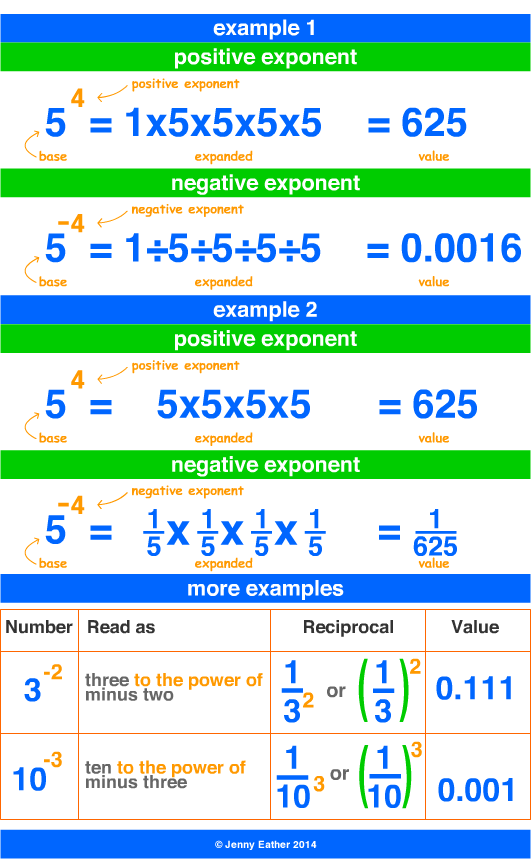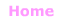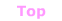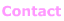Aa Bb Cc Dd Ee Ff Gg Hh Ii Jj Kk Ll Mm Nn Oo Pp Qq Rr Ss Tt Uu Vv Ww Xx Yy Zz

Nn

negative exponent

• an exponent is a small number placed
to the upper-right of a base number.
• a positive exponent shows the number
of times 1 is multiplied by the base.
• a negative exponent shows the number
of times 1 is divided by the base.
• a number having a negative exponent equals
its reciprocal with a positive exponent.

EXAMPLES:© Jenny Eather 2014. All rights reserved.